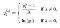# What is a Box Cox Transformation?

What is a Box Cox Transformation?

The Box-Cox transformation is a particularly useful family of transformations. It is defined as:where y^λ is the response variable and λ is the transformation parameter, For λ = 0, the natural log of the data is taken instead of using the above formula, here λ is a hyperparameter which has to be tuned according to the dataset

Many statistics tests and formulas are based on bell curves. Bell curves are considered “normal” for example. Normal being how most data looks prior to analysis. Box-cox transformations take data that isn’t “normal” and convert it into data that is easily used in formulas that require normal data. That is that fit into normal distribution sets like a bell curve or a similar progressions that can be easily plotted or used in statistical formulas.

The reasons to use a box-cox transformation are if you want to display your data, you’ll need to normalize it to make it easily consumed in plots and graphs. If you want to use most common statistical tools on the data, it will need to be normalized. If you want to use common statistical validation and comparison tools you’ll need to normalize the data.